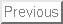1) (10 points)

Find the equation of the tangent line to the graph of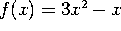at x=3.

2) (30 points)

Compute the following limits: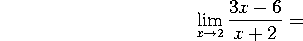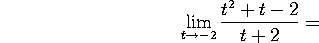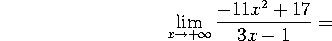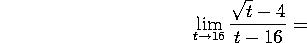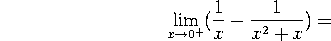3) (30 points)

Compute the derivative of the following functions. You don't need to simplify your answer: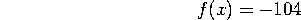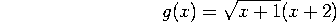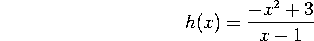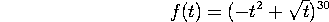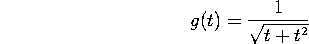4) (10 points)

The total profit (in dollars) from the sale of x espresso machines is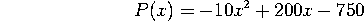Use the marginal profit to approximate the profit from the sale of the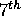espresso machine.

5) (20 points)

Let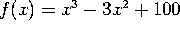.

a. Find the domain of f(x).

b. Compute f'(x) and find the critical points of f.

NOTE: You may choose to do a single chart for parts c and d.

c. Give the intervals where f is increasing and those where f is decreasing. Find all local maxima and all local minima.

d. Compute f''(x). Give the intervals where f is concave upward and those where f is concave downward. Is there any inflection point for f?

e. If f(x) for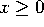represents the cost function of producing x hundred espresso machines, how many machines would you produce in order to minimize your cost?